Expansion of a vector: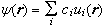A vector space is a collection of objects that can be added and multiplied by scalars. The operations called addition and multiplication are not necessarily our familiar algebraic operations, but they must obey certain rules.

Ordinary vectors in 3-dimensional space can be added using vector addition. Vector addition is different from ordinary addition, but it obeys the rules for the addition operation of a vector space.

In quantum mechanics, it is postulated that all possible states of a system form a vector space, i.e. they can be manipulated with two operations called addition and multiplication, which obey the rules for addition and multiplication in a vector space.  The operations are obviously different from the operations of adding and multiplying ordinary numbers.

Inner-product spaces are vector spaces for which an additional operation is defined, namely taking the inner product of two vectors. This operation associates which each pair of vectors a scalar, i.e. a number, not a vector. The operation also must obey certain rules, but again, as long as it does obey the rules it can be defined quite differently in different vector spaces. The vector space of ordinary 3-d vectors is an inner-product space; the inner product is the dot product.

The vector space that all possible states belong to in QM is not 3-dimensional, but infinite-dimensional. It is called a Hilbert space and it is an inner-product space. In Dirac notation the inner product of a vectors |ψ> with a vector |φ> is denoted by the symbol <ψ|φ>. This symbol denotes a number, not a vector. The inner product is quite different from ordinary multiplication, for example <φ|ψ> is not equal to <ψ|φ>, but the inner product satisfies the rule for an inner-product space.

In Dirac notation kets represent the vectors. To every ket corresponds exactly one bra. There is a one to one correspondence. |ψ> is a ket, the corresponding bra is <ψ|. If |x> is a ket, the corresponding bra is <x|.

The vectors in the Hilbert space can be represented in various representations, i.e. we can choose different bases, and give their components along the basis vectors. If we choose coordinate representation, the basis is the set of all vectors {|x>} and the component of a vector |ψ> along a vector |x> is given by the inner product <x|ψ> = ψ(x). If we evaluate ψ(x) for all |x> we get the wave function. Because we want to interpret the square of the wave function as a probability density, we require that the wave function can be normalized and that if we integrate the square of the normalized wave function over all space we get 1. The probability that we find the system somewhere in space is 1.

We require that the wave function is square-integrable. We therefore say that our Hilbert space is equivalent to the space of square-integrable functions.

#### Some functions such as ψ(x) = cos(kx) or ψ(x) = δ(x) are not square-integrable, the integral over all space always yields infinity. They therefore cannot represent real physical systems. But they are mathematically convenient, and we pretend they belong and treat them accordingly. The coordinate representation of the ket |x> is δ(x), the function is not square-integrable and |x> does not really belong to the Hilbert space. (|x> represents a system whose position is precisely known, and the uncertainty principle says that we cannot have this.) The bra <x| belongs to the dual space, because we can form <x|ψ> according to the rules of the inner product. (This integral is finite and yields a number). Therefore every good ket has a corresponding good bra, but not every good bra has a corresponding good ket. We generally do not worry about this. We just pretend that |x> is a good ket in the Hilbert space. The ket |p> represents a system with precisely defined momentum, which is also forbidden by the uncertainty principle. Its wave function <x|p> =h-1/2exp(i2πpx/h) is not square-integrable and strictly speaking |p> does not belong to the Hilbert space. Again, we generally ignore this and pretend that it belongs. Mathematical justification are tedious, but can be made.

A linear vector space V is a set of elements, {Vi}, which may be added and multiplied by scalars {αi} in such a way that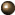the operation yields only elements of V (closure);addition and scalar multiplication obey the following rules: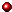i) Vi+Vj=Vj+Vi (commutativity);ii) Vi+(Vj+Vk)=(Vi+Vj)+Vk (associativity);iii) there exists a null vector, 0, in V such that 0+Vi=Vi+0=Vi;iv) for each vector Vi there exists an inverse (-Vi) in V such that Vi+(-Vi)=0 ;v) α(Vi+Vj)=αVi+αVj ;vi) (α+β)Vi=αVi+βVi ;vii) α(βVi)=(αβ)Vi .

The domain of allowed scalars is called the field F over which Vis defined. (Examples: F consists of all real numbers, or F consists of all complex numbers.)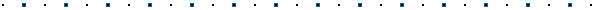#### Examples of vector spaces:Ordinary vectors in three-dimensional space;The set L2 of square integrable functions ψ(r,t) defined by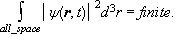A set of vectors {V1, V2, V3, ...} is linearly independent (LI) if there exists no linear relation of the form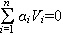, except for the trivial one with all αi =0.

A vector space is n-dimensional if it admits at most n LI vectors.  The space of ordinary vectors in three-dimensional space is 3-dimensional.  The space L2 is an infinite-dimensional vector space.

Given a set of n LI vectors in Vn, any other vector in V may be written as a linear combination of these.  The vectors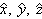are one example of a set of 3 LI vectors in 3 dimensions.  One can always choose such a set for every denumerably or non-denumerably infinite-dimensional vector space.  Any such set is called a basis that spans V.  The expansion coefficients are called the components of a vector in this basis.

Assume {ui(r), ui∈L2} forms a basis of L2.  Then every vector ψ in L2 may be written as,

the ci being the components of ψ(r) in this basis.

If all vectors are expanded in a given basis thento add vectors, add their components;to multiply a vector by α, multiply each component by α.

The inner product is a scalar function of two vectors satisfying the following rules:i) ≥0 ;ii) =* ;iii) .

Rule ii) and iii) combine to give <αVi+βVj|Vk>=α*<Vi|Vj>+β*<Vi|Vk>.

A vector space with an inner product is called an inner product space.  The inner product in L2 is defined by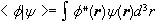.

The norm of a vector V defined by |V|=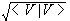.  A unit vector has norm 1.

Two vectors are orthogonal if their inner product vanishes.  A set of vectors {Vi} is called orthonormal if <Vi|Vj>ij.  Assume the vectors {ui(r)} are orthonormal and form a basis for L2.  Then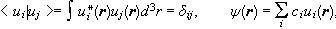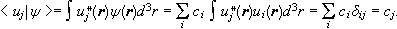The component cj is therefore equal to the scalar product of ui(r) and ψ(r).

Let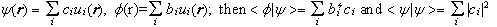.

The norm can be expressed in terms of the components.

The inner product obeys the Schwarz inequality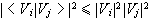.

The norm obeys the triangle inequality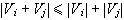.Hilbert SpaceLinear vector spaces in Quantum Mechanics#### Bases not belonging to V

It is sometimes convenient to introduce "bases" not belonging to V, but in terms of which any vector in V can nevertheless be expanded.

#### Examples:The set of functions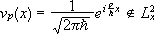may be considered a basis not belonging to L2x, labeled by the continuous index p. We write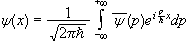,  or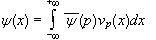. ψ(x) is an element of L2x.  {vp(x)}, the set of all plane waves with different values of p = hk, spans L2x.  Here p is a continuous index between -∞ and +∞ which labels the various functions in the set.  Every function in L2x can be expanded in one and only one way in terms of the vp(x);corresponds to the expansion coefficient ci in a discretely labeled basis.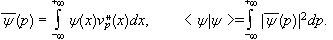The set {vp} is "orthonormalized in the Dirac sense".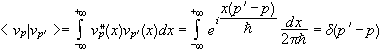.  (See Cohen-Tannoudji, appendix II, regarding the properties of the Dirac δ function.)  We have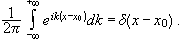If we define the δ function through the relationship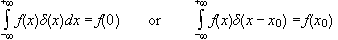, then δxo=δ(x-x0) may be considered a basis not belonging to L2x, labeled by the continuous index x0, which spans L2x.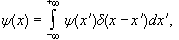where the expansion coefficient ψ(x') is given by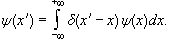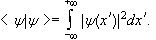The basis δ(x-x0) } is "orthonormalized in the Dirac sense".

#### Problem:

Find the Fourier transform of a δ function.Solution: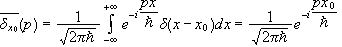by the definition of the Fourier transform.  In particular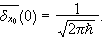The inverse Fourier transform then yields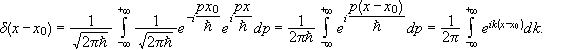This is an equivalent definition of the Dirac δ function.#### Dirac Notation

A vector is completely specified by its components in a given basis.  The same vector can be represented by distinct sets of components corresponding to different choices of bases. Dirac notation is a representation of a vector without an explicit choice of a basis.  Any element in V is called a ket vector or ket.  It is represented by the symbol | >, inside which there is a sign which distinguishes a given ket from all others.

#### Examples:An ordinary vector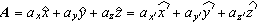in three dimensional space may be represented by the components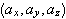or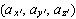depending on the choice of basis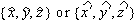.  But if we write A, we specify the vector without explicitly choosing a basis. In Dirac notation we would label this vector |A>.The quantum state of any physical system is characterized by a state vector, belonging to a space E, which is the state space of the system.  If ψ(r)>∈L2 then |ψ>∈E.  We may consider ψ(r) to be one specific representation of |ψ>, namely the set of components in a particular basis δ(r), r playing the role of an index.#### The Dual Space

A linear functional χ is a linear operation, which associates with each vector in V a scalar in the domain F.

|ψ> ∈ V implies that there exist a complex number χ, χ ∈ F.

&chi(λ1 | ψ1> + λ22>)= λ1(χ|ψ1>) + λ2(χ|ψ2>).

The set of all linear functionals defined on V forms a vector space, which is called the dual space of V, denoted by V*.  Forming the inner product <χ|ψ> of the vector |χ> with other elements |ψ> in V is a linear functional.  It associates with each vector |ψ> the complex number <χ|ψ>.  Therefore this operation is an element of the dual space V*.  We denote this element with the symbol <χ| and call it a bra vector or bra.  To every ket in V corresponds a bra in V*.  This correspondence is anti linear.

Take the ket λ11>+λ22>) = |φ>.  Form the inner product of this ket with any other vector |ψ> in V.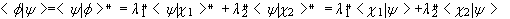.

The bra corresponding to |φ> is <φ|=l1*1|+λ2*2|.
We therefore have that the bra corresponding to λ|ψ> = |λψ > is <λψ|=λ* < ψ |.

Kets and bras are adjoints of each other.  To find the adjoint, take the complex conjugate of all scalars and replace each ket (bra) by its corresponding bra (ket).